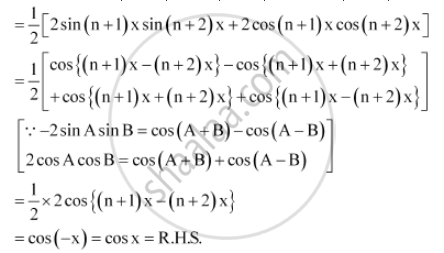CBSE (Arts) Class 11CBSE
Share

# Prove that Sin (N + 1)X Sin (N + 2)X + Cos (N + 1)X Cos (N + 2)X = Cos X - CBSE (Arts) Class 11 - Mathematics

ConceptTrigonometric Functions of Sum and Difference of Two Angles

#### Question

Prove that sin (n + 1)x sin (n + 2)x + cos (n + 1)x cos (n + 2)x = cos x

#### Solution

L.H.S. = sin (n + 1)x sin(n + 2)x + cos (n + 1)x cos(+ 2)xIs there an error in this question or solution?

#### APPEARS IN

NCERT Solution for Mathematics Textbook for Class 11 (2018 to Current)
Chapter 3: Trigonometric Functions
Q: 10 | Page no. 73

#### Video TutorialsVIEW ALL 

Solution Prove that Sin (N + 1)X Sin (N + 2)X + Cos (N + 1)X Cos (N + 2)X = Cos X Concept: Trigonometric Functions of Sum and Difference of Two Angles.
S